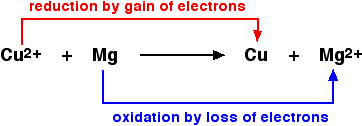# Definitions of Oxidation and Reduction

$$\newcommand{\vecs}{\overset { \rightharpoonup} {\mathbf{#1}} }$$ $$\newcommand{\vecd}{\overset{-\!-\!\rightharpoonup}{\vphantom{a}\smash {#1}}}$$$$\newcommand{\id}{\mathrm{id}}$$ $$\newcommand{\Span}{\mathrm{span}}$$ $$\newcommand{\kernel}{\mathrm{null}\,}$$ $$\newcommand{\range}{\mathrm{range}\,}$$ $$\newcommand{\RealPart}{\mathrm{Re}}$$ $$\newcommand{\ImaginaryPart}{\mathrm{Im}}$$ $$\newcommand{\Argument}{\mathrm{Arg}}$$ $$\newcommand{\norm}{\| #1 \|}$$ $$\newcommand{\inner}{\langle #1, #2 \rangle}$$ $$\newcommand{\Span}{\mathrm{span}}$$ $$\newcommand{\id}{\mathrm{id}}$$ $$\newcommand{\Span}{\mathrm{span}}$$ $$\newcommand{\kernel}{\mathrm{null}\,}$$ $$\newcommand{\range}{\mathrm{range}\,}$$ $$\newcommand{\RealPart}{\mathrm{Re}}$$ $$\newcommand{\ImaginaryPart}{\mathrm{Im}}$$ $$\newcommand{\Argument}{\mathrm{Arg}}$$ $$\newcommand{\norm}{\| #1 \|}$$ $$\newcommand{\inner}{\langle #1, #2 \rangle}$$ $$\newcommand{\Span}{\mathrm{span}}$$$$\newcommand{\AA}{\unicode[.8,0]{x212B}}$$

This page discusses the various definitions of oxidation and reduction (redox) in terms of the transfer of oxygen, hydrogen, and electrons. It also explains the terms oxidizing agent and reducing agent.

## Oxidation and reduction in terms of oxygen transfer

The terms oxidation and reduction can be defined in terms of the adding or removing oxygen to a compound. while this is not the most robust definition, as discussed below, it is the easiest to remember.

Oxidation and Reduction with respect to Oxygen Transfer

• Oxidation is the gain of oxygen.
• Reduction is the loss of oxygen.

For example, in the extraction of iron from its ore:Because both reduction and oxidation are occurring simultaneously, this is known as a redox reaction.

An oxidizing agent is substance which oxidizes something else. In the above example, the iron(III) oxide is the oxidizing agent. A reducing agent reduces something else. In the equation, the carbon monoxide is the reducing agent.

• Oxidizing agents give oxygen to another substance.
• Reducing agents remove oxygen from another substance.

## Oxidation and reduction in terms of hydrogen transfer

These are old definitions which are no longer used, except occasionally in organic chemistry.

Oxidation and Reduction with respect to Hydrogen Transfer

• Oxidation is the loss of hydrogen.
• Reduction is the gain of hydrogen.

Notice that these are exactly the opposite of the oxygen definitions (#1).

For example, ethanol can be oxidized to ethanal:An oxidizing agent is required to remove the hydrogen from the ethanol. A commonly used oxidizing agent is potassium dichromate(VI) solution acidified with dilute sulfuric acid. Ethanal can also be reduced back to ethanol by adding hydrogen. A possible reducing agent is sodium tetrahydridoborate, NaBH4. Again the equation is too complicated to consider at this point.More precise definitionsof oxidizing and reducing agents are

• Oxidizing agents add oxygen to another substance or remove hydrogen from it.
• Reducing agents remove oxygen from another substance or add hydrogen to it.

## Oxidation and reduction in terms of electron transfer

Oxidation and Reduction with respect to Electron Transfer

• Oxidation is loss of electrons
• Reduction is gain of electrons

Remembering these definitions is essential, and easily done using this convenient acronym:Example 1

The equation below shows an obvious example of oxygen transfer in a simple redox reaction:

$\ce{CuO + Mg \rightarrow Cu + MgO} \nonumber$

Copper(II) oxide and magnesium oxide are both ionic compounds. If the above is written as an ionic equation, it becomes apparent that the oxide ions are spectator ions. Omitting them gives:In the above reaction, magnesium reduces the copper(II) ion by transferring electrons to the ion and neutralizing its charge. Therefore, magnesium is a reducing agent. Another way of putting this is that the copper(II) ion is removing electrons from the magnesium to create a magnesium ion. The copper(II) ion is acting as an oxidizing agent.

## Summary

Confusion can result from trying to learn both the definitions of oxidation and reduction in terms of electron transfer and the definitions of oxidizing and reducing agents in the same terms. The following thought pattern can be helpful:

• An oxidizing agent oxidizes something else.
• Oxidation is loss of electrons (OIL RIG).
• Therefore, an oxidizing agent takes electrons from that other substance.
• Therefore, an oxidizing agent must gain electrons.

​Here is another mental exercise:

• An oxidizing agent oxidizes something else.
• Therefore, the oxidizing agent must be reduced.
• Reduction is gain of electrons (OIL RIG).
• Therefore, an oxidizing agent must gain electrons.

This page titled Definitions of Oxidation and Reduction is shared under a CC BY-NC 4.0 license and was authored, remixed, and/or curated by Jim Clark.